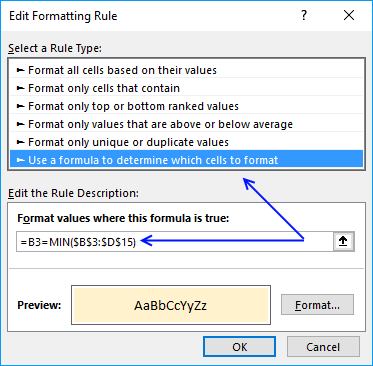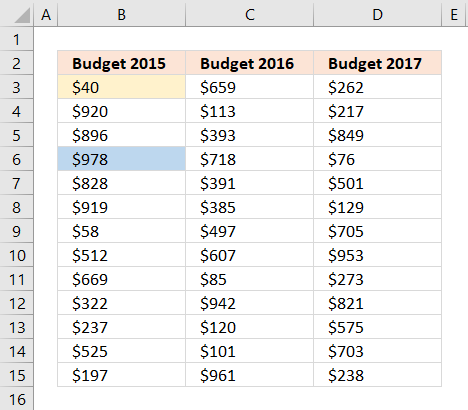Author: Oscar Cronquist Article last updated on November 17, 2017
Question: I have three columns and how do I identify the largest and smallest number? Where is the value?

I will now demonstrate four different formulas, all regular formulas that will tell you something about where the largest and smallest values are. I will also show you a technique that highlights the smallest and largest value.

The first formula returns the largest value from a cell range. The MAX function returns the largest number from a cell range.

Formula in cell C17:

=MAX(B3:D15)

The following formula returns the header name of the column that contains the largest value. I will explain these formulas later on, in this article.

Formula in cell C18:

=INDEX(\$B\$2:\$D\$2, ,SUMPRODUCT((\$B\$3:\$D\$15=MAX(B3:D15))* MATCH(COLUMN(\$B\$3:\$D\$15), COLUMN(\$B\$3:\$D\$15))))

This formula returns the address of the largest value, Excel has a grid with columns and rows. Example, address D16 is found in column D and row 16.

Formula in cell C19:

=ADDRESS(SUMPRODUCT((\$B\$3:\$D\$15=MAX(B3:D15))* ROW(\$B\$3:\$D\$15)), SUMPRODUCT((\$B\$3:\$D\$15=MAX(B3:D15))* COLUMN(\$B\$3:\$D\$15)))

The next formula creates a hyperlink that allows you to quickly move to and select the largest value. You simply click on the cell and Excel instantly navigates to that cell, even if it is on another worksheet.

Formula in cell C20:

=HYPERLINK("[Min and max out of multiple columns.xlsx]Sheet1!"&ADDRESS(SUMPRODUCT((\$B\$3:\$D\$15=MAX(B3:D15))* ROW(\$B\$3:\$D\$15)), SUMPRODUCT((\$B\$3:\$D\$15=MAX(B3:D15))* COLUMN(\$B\$3:\$D\$15))), MAX(B3:D15))

### Find the largest value in a cell range

I am now going to explaining the formula in cell C17: =MAX(B3:D15)

The MAX function returns the largest number in a cell range, you can also use multiple cell ranges, like this =MAX(B3:D15, F10:H22)

The MAX function allows you to have up to 255 arguments and it will ignore text and blank cells. However, if a cell contains an error value the function will also return an error value.

### How to identify the column header name for the maximum value

I am now going to explain the formula in cell C18.

#### Step 1 - Where is the largest value?

The following logical expression returns an array that displays the location of the maximum value.

\$B\$3:\$D\$15=MAX(B3:D15)

becomes

\$B\$3:\$D\$15=978

becomes

{40, 659, 262;920, 113, 217;896, 393, 849;978, 718, 76;828, 391, 501;919, 385, 129;58, 497, 705;512, 607, 953;669, 85, 273;322, 942, 821;237, 120, 575;525, 101, 703;197, 961, 238}=978

and returns {FALSE, FALSE, FALSE;FALSE, FALSE, FALSE;FALSE, FALSE, FALSE;TRUE, FALSE, FALSE;FALSE, FALSE, FALSE;FALSE, FALSE, FALSE;FALSE, FALSE, FALSE;FALSE, FALSE, FALSE;FALSE, FALSE, FALSE;FALSE, FALSE, FALSE;FALSE, FALSE, FALSE;FALSE, FALSE, FALSE;FALSE, FALSE, FALSE}

The picture below shows the array in cell range F3:H15, the corresponding location in the array returns TRUE. Remaining values in the array show FALSE.

#### Step 2 - Convert TRUE value to a column number

The next step is to multiply the array with the corresponding column numbers.

(\$B\$3:\$D\$15=MAX(B3:D15))* MATCH(COLUMN(\$B\$3:\$D\$15), COLUMN(\$B\$3:\$D\$15))

becomes

(\$B\$3:\$D\$15=MAX(B3:D15))* {1,2,3}

becomes

{FALSE, FALSE, FALSE;FALSE, FALSE, FALSE;FALSE, FALSE, FALSE;TRUE, FALSE, FALSE;FALSE, FALSE, FALSE;FALSE, FALSE, FALSE;FALSE, FALSE, FALSE;FALSE, FALSE, FALSE;FALSE, FALSE, FALSE;FALSE, FALSE, FALSE;FALSE, FALSE, FALSE;FALSE, FALSE, FALSE;FALSE, FALSE, FALSE}* {1,2,3}

and returns {0,0,0;0,0,0;0,0,0;1,0,0;0,0,0;0,0,0;0,0,0;0,0,0;0,0,0;0,0,0;0,0,0;0,0,0;0,0,0}

The picture below displays the array in cell range F3:H15, The TRUE value now contains number 1 which is the relative column number in cell range B3:D15 for that location. In other words, the maximum value is found in the first column.

#### Step 3 - Sum values in array

The SUMPRODUCT function lets you do these mathematical operations without the need to enter the formula as an array formula.

SUMPRODUCT((\$B\$3:\$D\$15=MAX(B3:D15))* MATCH(COLUMN(\$B\$3:\$D\$15), COLUMN(\$B\$3:\$D\$15)))

becomes

SUMPRODUCT({0,0,0;0,0,0;0,0,0;1,0,0;0,0,0;0,0,0;0,0,0;0,0,0;0,0,0;0,0,0;0,0,0;0,0,0;0,0,0})

and returns 1.

#### Step 4 - Return header name

The INDEX function fetches a value based on coordinates. Since the values are in a single row we need only to enter the argument for the column number.

INDEX(cell_ref, row_number, column_number)

INDEX(\$B\$2:\$D\$2, ,SUMPRODUCT((\$B\$3:\$D\$15=MAX(B3:D15))* MATCH(COLUMN(\$B\$3:\$D\$15), COLUMN(\$B\$3:\$D\$15))))

becomes

INDEX(\$B\$2:\$D\$2, ,1)

becomes

INDEX({"Budget 2015","Budget 2016","Budget 2017"}, ,1)

and returns Budget 2015 in cell C18.

### Calculate the address of the maximum value

Allow me to explain the formula in cell C19. The ADDRESS function returns a cell reference as a text value, to be able to do that it needs to know the row and column number.

If you read the previous explanation for the formula in cell C18, you know how to calculate which column the value is in. We can do the same calculation, with minor changes to the formula, to calculate the row number.

ADDRESS(SUMPRODUCT((\$B\$3:\$D\$15=MAX(B3:D15))* ROW(\$B\$3:\$D\$15)), SUMPRODUCT((\$B\$3:\$D\$15=MAX(B3:D15))* COLUMN(\$B\$3:\$D\$15)))

becomes

and returns \$B\$6 in cell C19.

### Create a clickable link that navigates to the maximum value

This section explains the formula in cell C20. The HYPERLINK function allows you to create a link to a cell location. (It can do more than that but this is all you need to know for now.)

The link location is a cell reference to a specific cell in your workbook. You need to specify the workbook name, worksheet name and the cell address. Read the previous explanation to learn how to calculate the cell reference of the maximum value.

HYPERLINK("[Min and max out of multiple columns.xlsx]Sheet1!"&ADDRESS(SUMPRODUCT((\$B\$3:\$D\$15=MAX(B3:D15))* ROW(\$B\$3:\$D\$15)), SUMPRODUCT((\$B\$3:\$D\$15=MAX(B3:D15))* COLUMN(\$B\$3:\$D\$15))), MAX(B3:D15))

becomes

HYPERLINK("[Min and max out of multiple columns.xlsx]Sheet1!"&B6, MAX(B3:D15))

The second argument is the value that you want the cell to show, in this case the maximum value.

HYPERLINK("[Min and max out of multiple columns.xlsx]Sheet1!"&B6, MAX(B3:D15))

becomes

HYPERLINK("[Min and max out of multiple columns.xlsx]Sheet1!"&B6, 978)

and returns 978 (hyperlink) in cell C20.

### How to easily spot the largest and smallest value

Conditional formatting allows you to format specific values using a formula or built-in criteria, in this case, I will highlight the cells containing the maximum and minimum value in a cell range.

1. Select cell range B3:D15
2. Go to tab "Home" if you are not already there
3. Click on "Conditional formatting" button
4. Click "New Rule.."5. Click on "Use a formula to determine which cells to format"
6. Type this formula in field "Format values where this formula is true:": =B3=MAX(\$B\$3:\$D\$15)
7. Click "Format..." button
8. Click on tab "Fill"
9. Pick a color
10. Click "OK" button
11. Click "OK" button

Repeat above steps to highlight the minimum value using this formula: =B3=MIN(\$B\$3:\$D\$15)
Remember to pick a different color.Min and max out of multiple columns.xlsx